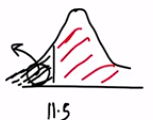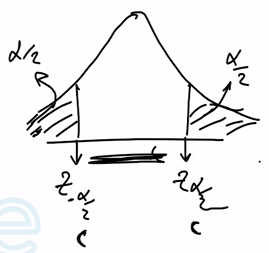Need Help?

Subscribe to Probability

###### \${selected_topic_name}
• Notes

A textile fiber manufacturer is investigating a new
drapery yarn, which the company claims has a mean thread
elongation of 12 kilograms with a standard deviation of 0.5
kilograms. The company wishes to test the hypothesis
$H_{0} : \mu=12$ against $H_{1} : \mu<12,$ using a random sample of
four specimens.

(a) What is the type I error probability if the critical region is
defined as $\overline{x}<11.5$ kilograms?
(b) Find $\beta$ for the case where the true mean elongation is
11.25 kilograms.
(c) calculate the $P$ -value if the observed statistic is
(a) $\overline{x}=11.25 \quad$ (b) $\overline{x}=11.0 \quad$ (c) $\overline{x}=11.75$

$\mu=12 \mathrm{kg}$

$\sigma=0.5$

$H_{0} : \mu=12$ against $H_{1} : \mu<12$

$n=4$(a) $\overline{x}<11.5$

$\alpha=P(\overline{x}<11.5)=P\left(\frac{\overline{x}-\mu}{\sigma / \sqrt{n}} \leq \frac{11.5-12}{\frac {0.5}{\sqrt 4}}\right)$

$=p(Z \leq -2)$
$=0.02275$

(b) $\beta$ when $\mu=11.25$

$\beta=P\left(\text { accept } H_{0}\right)=P(\overline{x}>11.5)$

$=P\left(Z>\frac{11.5 - 11.25}{\frac {0.5}{\sqrt 4}}\right)$

$\beta=P(Z>1)=1-P(Z<1)$

$=0.15866$

(c) $P=\phi\left(Z_{0}\right)$

(a) $P=\phi\left(\frac{11.25-12}{0.5 / \sqrt{4}}\right)=\phi (-3)$

$=0.00135$

(b) $P=\phi\left(\frac{11-12}{\frac {0.5}{\sqrt{4}}}\right)=\phi (-4)=0$

(c) $P=\phi \left(\frac{11.75-12}{0.5 / \sqrt{4}}\right)=\phi(-1)$

$=0.15866$

A manufacturer is interested in the output voltage of a
power supply used in a PC. Output voltage is assumed to be
normally distributed, with standard deviation 0.25 Volts, and
the manufacturer wishes to test $H_{0} : \mu=5$ Volts against
$H_{1} : \mu \neq 5$ Volts, using $n=8$ units.

(a) The acceptance region is $4.85 \leq \overline{x} \leq 5.15$ . Find the value
of $\alpha .$
(b) Find the power of the test for detecting a true mean output
voltage of 5.1 Volts.
(c) suppose that the manu facturer wants the type I error
probability for the test to be $\alpha=0.05 .$ Where should
the acceptance region be located?

$\sigma=0.25 \mathrm{v} \quad \mathrm{n}=8$

$H_{0}: \mu=5 v$
$H_{1} : \mu \neq 5$

(a) $4.85 \leq \overline{x} \leq 5.15$

$\alpha ?!$

$\alpha=P(\overline{x}<4.85)+P(\overline{x}>5.15)$

$P\left(Z <\frac{4.85-5}{0.25 /\sqrt 8}\right)+\left(Z>\frac{{5.15}-5}{0.25/\sqrt{8}}\right)$

$=P(Z<-1.7)+P(Z>1.7)$

$\alpha = 0.08914$

(b) $\mu=5.1,$ The Power

Power $=1- \beta$

$\beta=P(4.85 \leq \overline x \leq 5.15)$

$=P\left(\frac{4.85-5.1}{0.25/\sqrt 8} \leq Z \leq \frac{5.15 -5.1}{\frac{0.25}{\sqrt{8}}}\right)$

$=P(-2.83 \leq Z \leq 0.566)$

$=P(Z \leq 0.566)-P(Z \leq -2.83)$

$=0.71566-0.00233$

$=0.71333$

Power $=1-\beta=0.2867$(c) $\alpha=0.05$

$\frac{\alpha}{2}=0.025$

$0.025=P\left(Z \leq \frac{c-5}{0.25 / \sqrt{8}}\right)$

$Z=\pm 1.96$

$\frac{c-5}{0.25 / \sqrt{8}}=-1.96 \rightarrow c=4.83$

$=1.96 \rightarrow c=5.17$

$(4.83\leq \overline{x} \leq 5.17)$